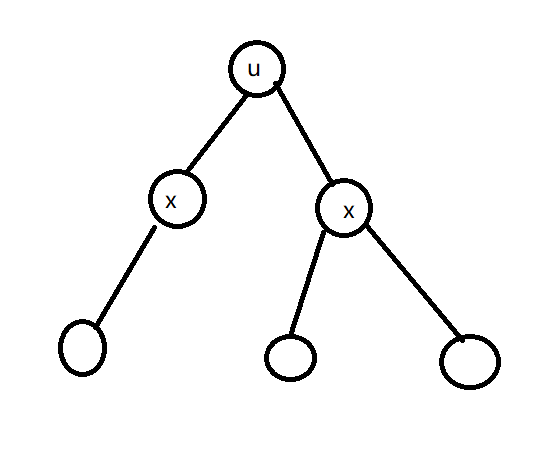# 牛客算法周周练13 B - 病毒感染 换根dp

https://ac.nowcoder.com/acm/contest/6173/B

2 1
1 2


1 2



(图的类型保证没有大小大于等于3的环)

dp[u] 表示u点到所有其他所有点的最短距离之和

1. 第一次dfs的时候从下向上传递 ， 此时dp[u]表示u点到子树中所有点的最短距离之和 ， x节点对当前节点的贡献是 dp[x] + size[x] , 其含义在孩子节点x的基础上面，所有的子节点全部深度全部加一 ， 也就是 + size[x]
2. 从上到下传递的时候，当前节点u对孩子节点的贡献是 dp[u] - dp[x] - size[x] + size[u] - size[x] ，解释： 先将当前节点的dp[u] 剪掉 所要传孩子节点x的dp[x] 贡献删掉， 根据第一步 ， 其贡献是dp[x] + size[x] , size[u] 要减掉size[x] , 这两个dp[u] - dp[x] - size[x] , 和 size[u] - size[x] , 都相当于在下传贡献的时候， 先将孩子节点的贡献删掉 , 然后将孩子节点数量改变一下，因为下一次x节点就变成了根节点 ， dp值要变， size孩子节点也要变， 之后要用到。#include <iostream>
#include <cstdio>
#include <algorithm>
#include <unordered_map>
#include <vector>
#include <map>
#include <list>
#include <queue>
#include <cstring>
#include <cstdlib>
#include <ctime>
#include <cmath>
#include <stack>
#include <set>
#pragma GCC optimize(3 , "Ofast" , "inline")
using namespace std ;
#define ios ios::sync_with_stdio(false) , cin.tie(0) , cout.tie(0)
#define x first
#define y second
typedef long long ll ;
const double esp = 1e-6 , pi = acos(-1) ;
typedef pair<int , int> PII ;
const int N = 1e6 + 10 , INF = 0x3f3f3f3f , mod = 1e9 + 7;
ll in()
{
ll x = 0 , f = 1 ;
char ch = getchar() ;
while(!isdigit(ch)) {if(ch == '-') f = -1 ; ch = getchar() ;}
while(isdigit(ch)) x = x * 10 + ch - 48 , ch = getchar() ;
return x * f ;
}
vector<int> v[N] ;
int size[N] , dp[N] , n , m ;
void dfs(int u , int f)
{
size[u] = 1 ;
for(auto x : v[u])
{
if(x == f) continue ;
dfs(x , u) ;
size[u] += size[x] ;
dp[u] += dp[x] + size[x] ;
}
return ;
}
void dfs1(int u , int f , int sum , int res)
{
dp[u] += sum ;
size[u] += res ;
for(auto x : v[u])
{
if(x == f) continue ;
dfs1(x , u , dp[u] - dp[x] - size[x] + size[u] - size[x] , size[u] - size[x]) ;
}
return ;
}
int main()
{
n = in() , m = in() ;
for(int i = 1 , a , b ; i <= m ;i ++ )
a = in() , b = in() , v[a].push_back(b) , v[b].push_back(a) ;
dfs(1 , 0) ;
dfs1(1 , 0 , 0 , 0) ;
int ans = INF ;
for(int i = 1; i <= n ;i ++ )
ans = min(ans , dp[i]) ;
for(int i = 1; i <= n ;i ++ )
if(dp[i] == ans)
cout << i << " " ;
puts("") ;
return 0 ;
}
/*
9 8
1 2
2 3
3 5
3 6
1 4
4 7
4 8
7 9
*/



07-0158

#### 牛客算法周周练1310-21319

#### 树的重心

06-301143

#### 牛客算法周周练13 （A 水 B 换根dp C 水 D 水 E dp）

06-30721

#### 牛客算法周周练13水题

10-21280

#### 牛客网小白月赛8

04-15137

#### 初级玩转Linux+Ubuntu(嵌入式开发基础课程)

06-26

06-103万+

#### 我以为我对Mysql事务很熟，直到我遇到了阿里面试官

06-191万+

#### MFC一站式终极全套课程包

09-28©️2020 CSDN 皮肤主题: 书香水墨 设计师: CSDN官方博客点击重新获取扫码支付1.余额是钱包充值的虚拟货币，按照1:1的比例进行支付金额的抵扣。
2.余额无法直接购买下载，可以购买VIP、C币套餐、付费专栏及课程。余额充值